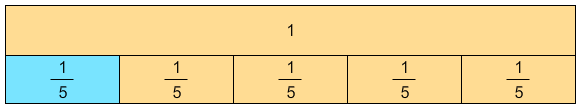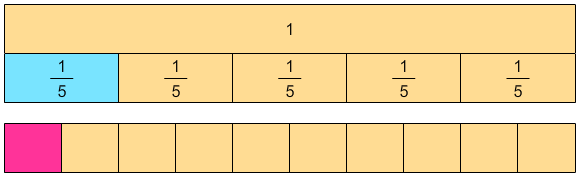Dividing Unit Fractions by Whole Numbers

Rate 0 stars Common Core
Quiz size:
Message preview:
Someone you know has shared Dividing Unit Fractions by Whole Numbers quiz with you:

To play this quiz, click on the link below:

https://www.turtlediary.com/quiz/dividing-unit-fractions-by-whole-numbers.html

Hope you have a good experience with this site and recommend to your friends too.

Login to rate activities and track progress.
Login to rate activities and track progress.

Let's find the quotient of:

 1 5
÷  2

First of all, make a model of fraction
 1 5
.To divide
 1 5
by 2, divide each segment of all
 1 5
parts into two equal parts.Since, there are 10 equal parts of the whole, each part will represent
 1 10
.
So,
 1 5
÷  2 =
 1 10
.

ds

A B C D E F G H I J K L M N O P Q R S T U V W X Y Z

Help

Remember :

The smallest number is the one that comes first while counting.

Solution :

To arrange the given numbers in order from smallest to greatest, find the smallest number among all the given numbers.

21,27,23

21 is the smallest number.